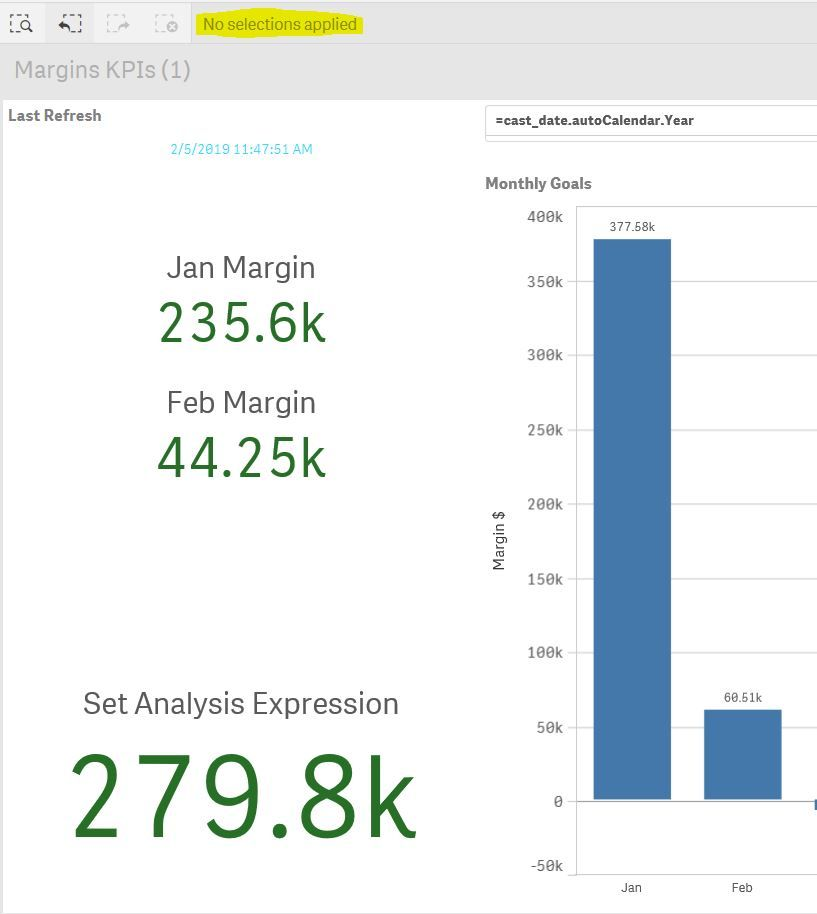# App Development

Announcements
Read about the latest Qlik Community enhancements on the Community News blog!
cancel
Showing results for
Did you mean:Contributor III

## Set Analysis in Bar Chart Not Working

All,

I am trying to create a bar chart that has Months as the Dimension and Margin \$ in the measures as a Set Analysis expression.  My Set Analysis expression gives the YTD Margin \$ for 2019.

My issue is that the resulting bar chart numbers are inaccurate until I use a filter to select the Year = 2019...

Here is my Set Analysis Expression:

(SUM(AGGR((SUM({<[cast_date.autoCalendar.YearsAgo]={0}>}amount_sold)+
SUM({<[cast_date.autoCalendar.YearsAgo]={0}>}prdct_cst*quantity_returned)+
SUM(AGGR(IF(Credit_Card_Accepted__c='Yes',(((IF(SUM({<[cast_date.autoCalendar.YearsAgo]={0}>}amount_sold>0),SUM({<[cast_date.autoCalendar.YearsAgo]={0}>}prdct_cst*quantity_ordered),0)))*.02),0),asin))-
IF(SUM({<[cast_date.autoCalendar.YearsAgo]={0}>}amount_sold>0),SUM({<[cast_date.autoCalendar.YearsAgo]={0}>}prdct_cst*quantity_ordered),0)-
SUM({<[cast_date.autoCalendar.YearsAgo]={0}>}quantity_ordered*prdct_fee)-
(SUM({<[cast_date.autoCalendar.YearsAgo]={0}>}(quantity_ordered)*est_ref_fee)-SUM({<[cast_date.autoCalendar.YearsAgo]={0}>}(quantity_returned)*est_ref_fee))-
(SUM({<[cast_date.autoCalendar.YearsAgo]={0}>}quantity_returned)*5)-
SUM(AGGR(IF(Free_Shipping__c='Yes',0,IF(Direct_Ship__c='Yes',0,
(IF(Standard_Shipping_Method__c='Small Parcel',SUM(Small_Parcel_Cost_Per_Pound__c)*(SUM({<[cast_date.autoCalendar.YearsAgo]={0}>}quantity_ordered)-Sum({<[cast_date.autoCalendar.YearsAgo]={0}>}quantity_returned)),
IF(Standard_Shipping_Method__c='LTL Freight',SUM(LTL_Freight_Cost_Per_Pound__c)*(SUM({<[cast_date.autoCalendar.YearsAgo]={0}>}quantity_ordered)-Sum({<[cast_date.autoCalendar.YearsAgo]={0}>}quantity_returned)),
IF(Standard_Shipping_Method__c='International',SUM(International_Cost_Per_Pound__c)*(SUM({<[cast_date.autoCalendar.YearsAgo]={0}>}quantity_ordered)-Sum({<[cast_date.autoCalendar.YearsAgo]={0}>}quantity_returned)),)))))),asin))-
(SUM(AGGR(((SUM(Small_Parcel_Cost_Per_Pound__c)*(SUM({<[cast_date.autoCalendar.YearsAgo]={0}>}quantity_ordered)-Sum({<[cast_date.autoCalendar.YearsAgo]={0}>}quantity_returned)))*0.75),asin)))-
(IF(SUM({<[cast_date.autoCalendar.YearsAgo]={0}>}amount_sold>0),SUM({<[cast_date.autoCalendar.YearsAgo]={0}>}prdct_cst*quantity_ordered),0)*0.01)-
SUM({<[cast_date.autoCalendar.YearsAgo]={0}>}amount_returned)),[cast_date.autoCalendar.Month])))

The two screenshots below show this error along with KPIs to show the correct numbers.  The 1st screenshot shows the Bar Chart numbers incorrectly with no selection applied while the 2nd screenshot shows the Bar Chart numbers correctly while filtering:Unfiltered = IncorrectPlease let me know if I need to change my expression in order to get the desired results.  Thanks.

Labels (3)

• ### Set Analysis

5 RepliesMVP

Not entirely sure, What is the purpose you used Sum(aggr(Expression)) around your measure?

Try this way?

(SUM(AGGR(NODISTINCT (SUM({<[cast_date.autoCalendar.YearsAgo]={0}>}amount_sold)+
SUM({<[cast_date.autoCalendar.YearsAgo]={0}>}prdct_cst*quantity_returned)+
SUM(AGGR(IF(Credit_Card_Accepted__c='Yes',(((IF(SUM({<[cast_date.autoCalendar.YearsAgo]={0}>}amount_sold>0),SUM({<[cast_date.autoCalendar.YearsAgo]={0}>}prdct_cst*quantity_ordered),0)))*.02),0),asin))-
IF(SUM({<[cast_date.autoCalendar.YearsAgo]={0}>}amount_sold>0),SUM({<[cast_date.autoCalendar.YearsAgo]={0}>}prdct_cst*quantity_ordered),0)-
SUM({<[cast_date.autoCalendar.YearsAgo]={0}>}quantity_ordered*prdct_fee)-
(SUM({<[cast_date.autoCalendar.YearsAgo]={0}>}(quantity_ordered)*est_ref_fee)-SUM({<[cast_date.autoCalendar.YearsAgo]={0}>}(quantity_returned)*est_ref_fee))-
(SUM({<[cast_date.autoCalendar.YearsAgo]={0}>}quantity_returned)*5)-
SUM(AGGR(IF(Free_Shipping__c='Yes',0,IF(Direct_Ship__c='Yes',0,
(IF(Standard_Shipping_Method__c='Small Parcel',SUM(Small_Parcel_Cost_Per_Pound__c)*(SUM({<[cast_date.autoCalendar.YearsAgo]={0}>}quantity_ordered)-Sum({<[cast_date.autoCalendar.YearsAgo]={0}>}quantity_returned)),
IF(Standard_Shipping_Method__c='LTL Freight',SUM(LTL_Freight_Cost_Per_Pound__c)*(SUM({<[cast_date.autoCalendar.YearsAgo]={0}>}quantity_ordered)-Sum({<[cast_date.autoCalendar.YearsAgo]={0}>}quantity_returned)),
IF(Standard_Shipping_Method__c='International',SUM(International_Cost_Per_Pound__c)*(SUM({<[cast_date.autoCalendar.YearsAgo]={0}>}quantity_ordered)-Sum({<[cast_date.autoCalendar.YearsAgo]={0}>}quantity_returned)),)))))),asin))-
(SUM(AGGR(((SUM(Small_Parcel_Cost_Per_Pound__c)*(SUM({<[cast_date.autoCalendar.YearsAgo]={0}>}quantity_ordered)-Sum({<[cast_date.autoCalendar.YearsAgo]={0}>}quantity_returned)))*0.75),asin)))-
(IF(SUM({<[cast_date.autoCalendar.YearsAgo]={0}>}amount_sold>0),SUM({<[cast_date.autoCalendar.YearsAgo]={0}>}prdct_cst*quantity_ordered),0)*0.01)-
SUM({<[cast_date.autoCalendar.YearsAgo]={0}>}amount_returned)),[cast_date.autoCalendar.Month])))

Before develop something, think If placed (The Right information | To the right people | At the Right time | In the Right place | With the Right context)MVP

Try this

```=(SUM(AGGR((SUM({<[cast_date.autoCalendar.YearsAgo]={0}>}amount_sold)+
SUM({<[cast_date.autoCalendar.YearsAgo]={0}>}prdct_cst*quantity_returned)+
SUM(AGGR(IF(Credit_Card_Accepted__c='Yes',(((IF(SUM({<[cast_date.autoCalendar.YearsAgo]={0}>}amount_sold>0),SUM({<[cast_date.autoCalendar.YearsAgo]={0}>}prdct_cst*quantity_ordered),0)))*.02),0),asin))-
IF(SUM({<[cast_date.autoCalendar.YearsAgo]={0}>}amount_sold>0),SUM({<[cast_date.autoCalendar.YearsAgo]={0}>}prdct_cst*quantity_ordered),0)-
SUM({<[cast_date.autoCalendar.YearsAgo]={0}>}quantity_ordered*prdct_fee)-
(SUM({<[cast_date.autoCalendar.YearsAgo]={0}>}(quantity_ordered)*est_ref_fee)-SUM({<[cast_date.autoCalendar.YearsAgo]={0}>}(quantity_returned)*est_ref_fee))-
(SUM({<[cast_date.autoCalendar.YearsAgo]={0}>}quantity_returned)*5)-
SUM(AGGR(IF(Free_Shipping__c='Yes',0,IF(Direct_Ship__c='Yes',0,
(IF(Standard_Shipping_Method__c='Small Parcel',SUM(Small_Parcel_Cost_Per_Pound__c)*(SUM({<[cast_date.autoCalendar.YearsAgo]={0}>}quantity_ordered)-Sum({<[cast_date.autoCalendar.YearsAgo]={0}>}quantity_returned)),
IF(Standard_Shipping_Method__c='LTL Freight',SUM(LTL_Freight_Cost_Per_Pound__c)*(SUM({<[cast_date.autoCalendar.YearsAgo]={0}>}quantity_ordered)-Sum({<[cast_date.autoCalendar.YearsAgo]={0}>}quantity_returned)),
IF(Standard_Shipping_Method__c='International',SUM(International_Cost_Per_Pound__c)*(SUM({<[cast_date.autoCalendar.YearsAgo]={0}>}quantity_ordered)-Sum({<[cast_date.autoCalendar.YearsAgo]={0}>}quantity_returned)),)))))),asin))-
(SUM(AGGR(((SUM(Small_Parcel_Cost_Per_Pound__c)*(SUM({<[cast_date.autoCalendar.YearsAgo]={0}>}quantity_ordered)-Sum({<[cast_date.autoCalendar.YearsAgo]={0}>}quantity_returned)))*0.75),asin)))-
(IF(SUM({<[cast_date.autoCalendar.YearsAgo]={0}>}amount_sold>0),SUM({<[cast_date.autoCalendar.YearsAgo]={0}>}prdct_cst*quantity_ordered),0)*0.01)-
SUM({<[cast_date.autoCalendar.YearsAgo]={0}>}amount_returned)),[cast_date.autoCalendar.Month], [cast_date.autoCalendar.Year])))```Contributor III
Author
Didn't work, same result.Contributor III
Author
Did not workMVP

Would you be able to share a sample to see the issue?Tags
Community Browser